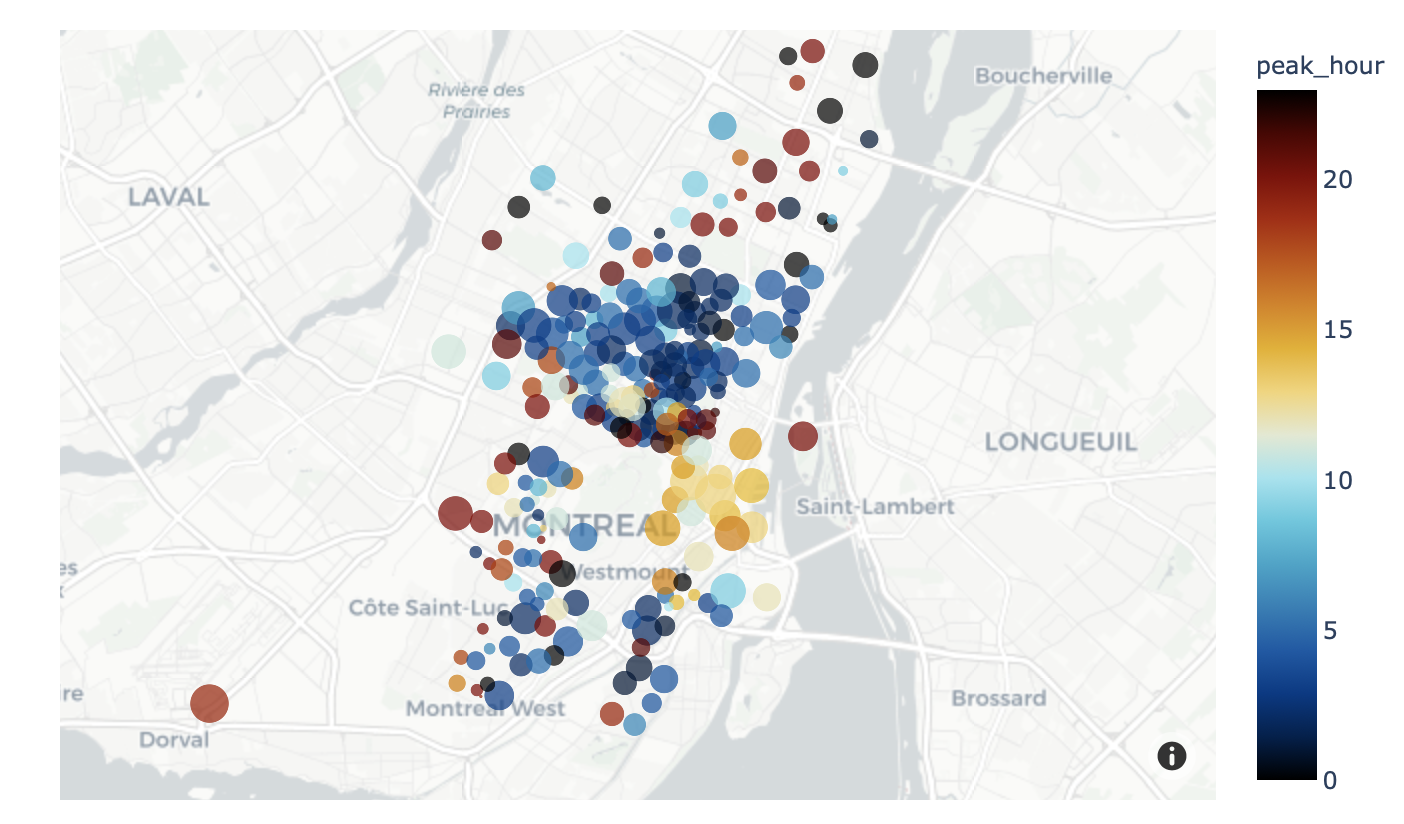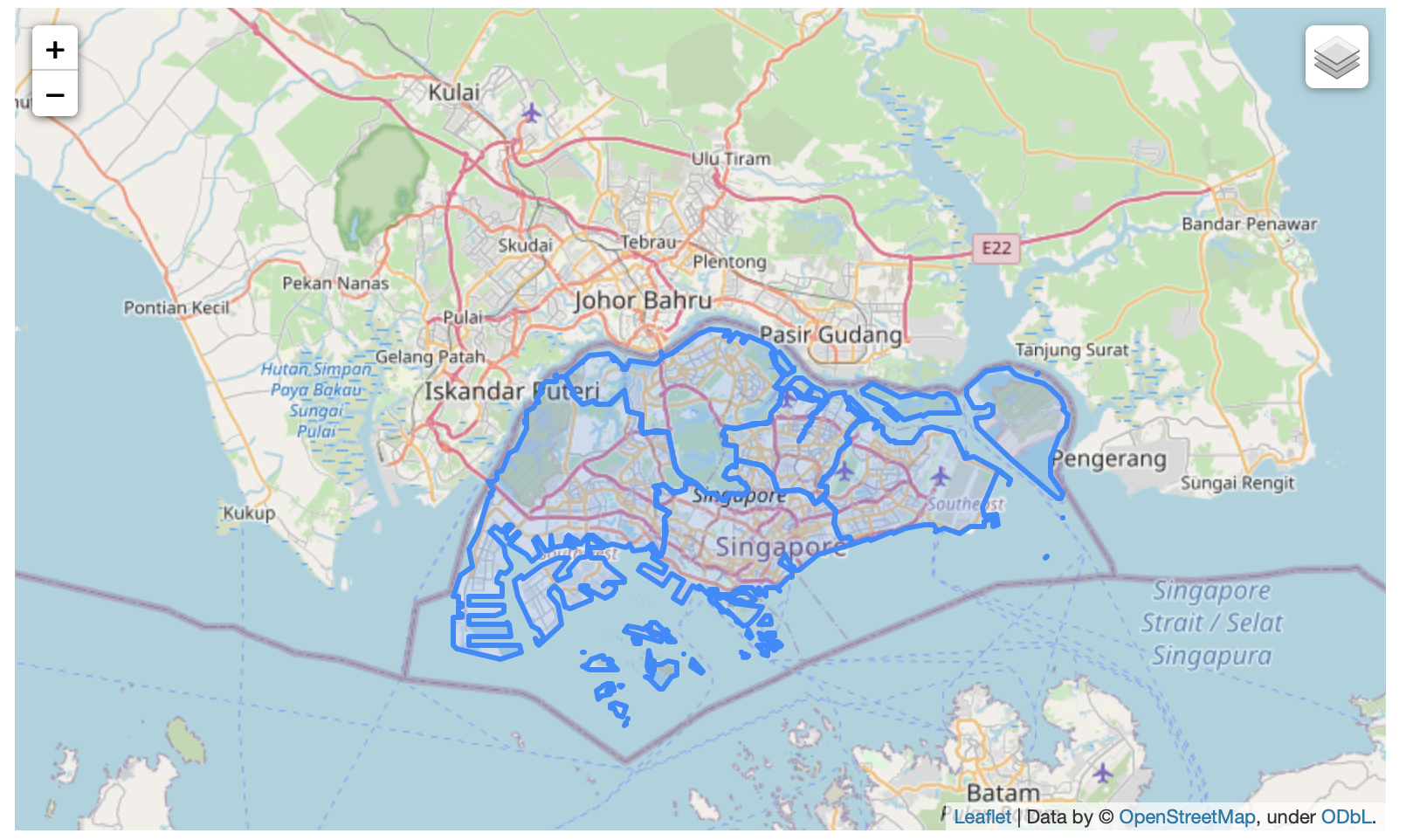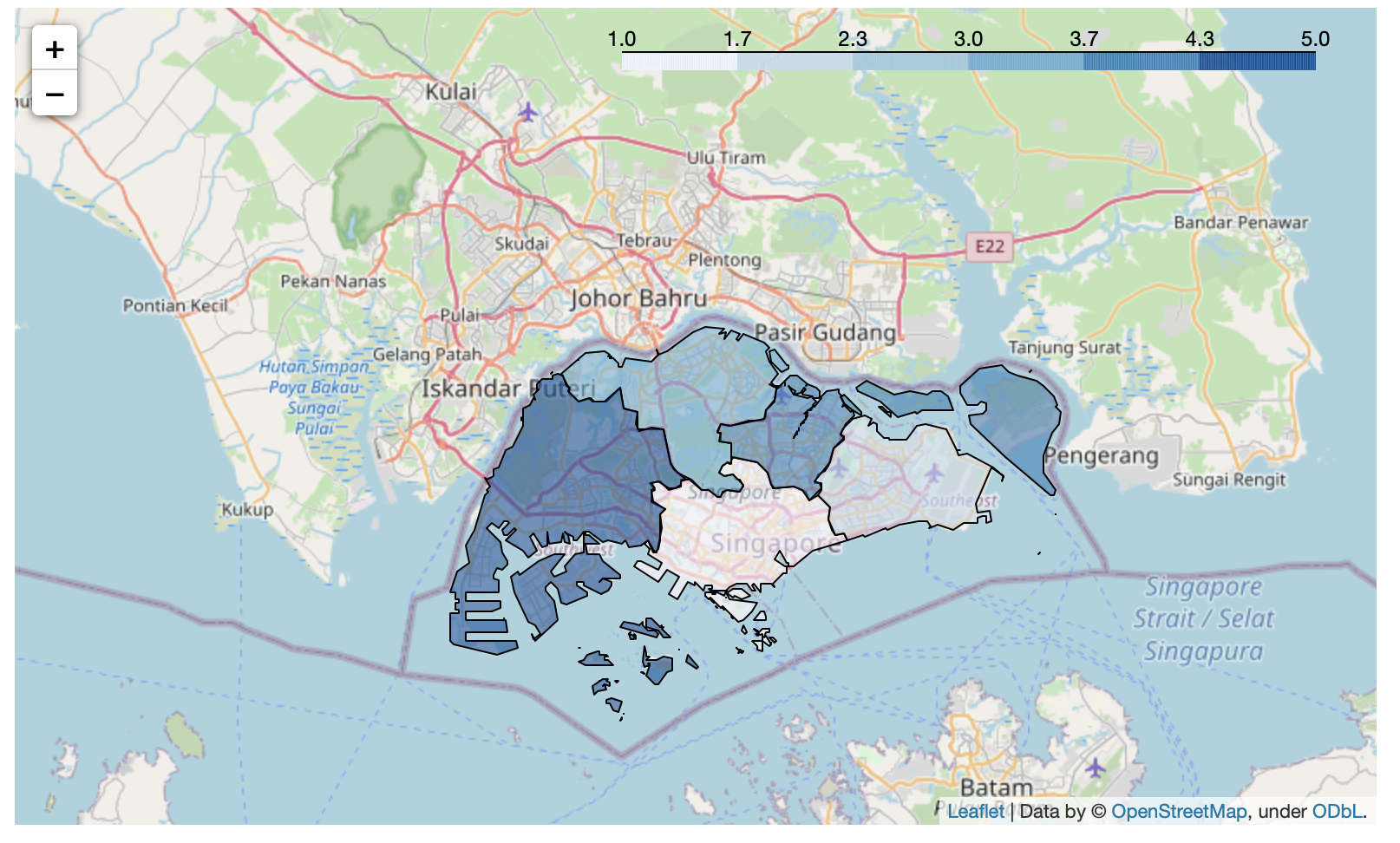# GPS plots

04 Sep 2020

A million years since i’ve been here!!

Today’s interesting topic of the day - GPS plots!

Plotly and folium have made things so much easier these days - no tokens whatsoever required please

below are mostly from the documentation pages - just extracting the critical bits so its easier for everyone!

1) Doing a scatterplot of points on a map``````import plotly.express as px
df = px.data.carshare()
fig = px.scatter_mapbox(df,
lat="centroid_lat",
lon="centroid_lon",
color="peak_hour",
size="car_hours",
color_continuous_scale=px.colors.cyclical.IceFire,
mapbox_style="carto-positron",
size_max=15,
zoom=10)
fig.show()
``````

You can also use `fig.write_html("file.html")` instead of show to write to an interactive html file!

Change “carto-positron” to “open-street-map” to use maps from OSM! Full list of free raster tiles here: https://plotly.com/python/mapbox-layers/

2) Drawing polygons on a mapYou can download a geojson from here (full credits to the owner!): https://raw.githubusercontent.com/yinshanyang/singapore/master/maps/1-region.geojson

``````import geopandas as gpd
import folium

# plot
m = folium.Map(location=[1.4, 103.8], zoom_start=10)

folium.GeoJson(gdf

m
``````

3) Drawing polygons on a map with additional information as colour``````import pandas as pd
import geopandas as gpd
import folium

df = pd.DataFrame([
['CENTRAL REGION', 1],
['EAST REGION', 2],
['NORTH REGION', 3],
['NORTH-EAST REGION', 4],
['WEST REGION', 5],
], columns=['name', 'display_color'])

m = folium.Map(location=[1.4, 103.8], zoom_start=10)

folium.Choropleth(geo_data='1-region.geojson', data=df,
columns=['name', 'display_name'],
key_on='feature.properties.name',